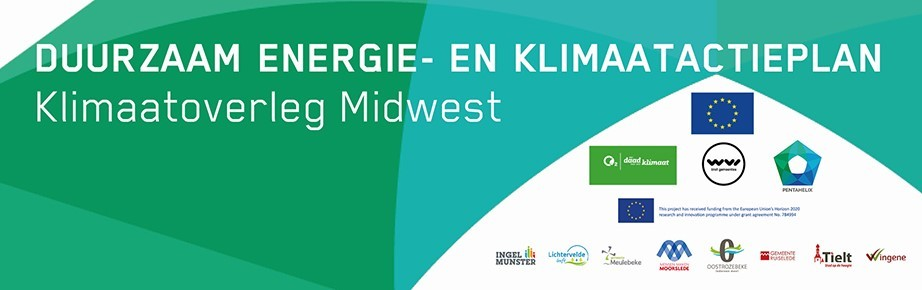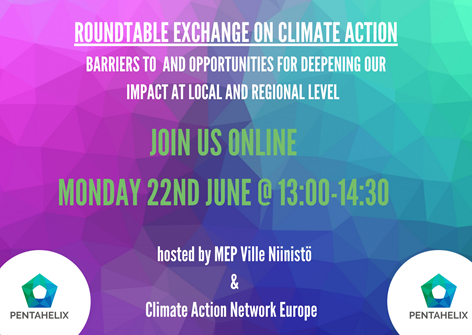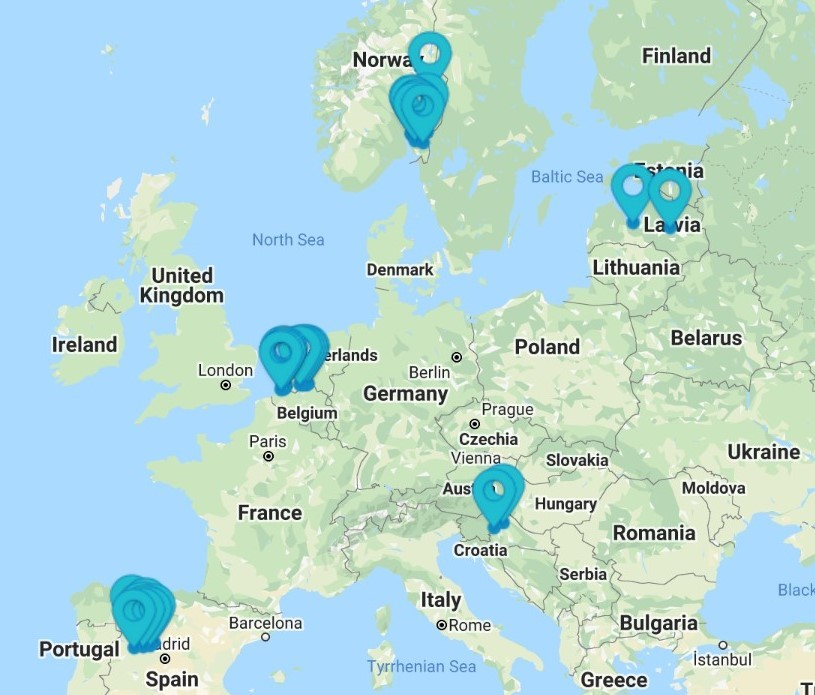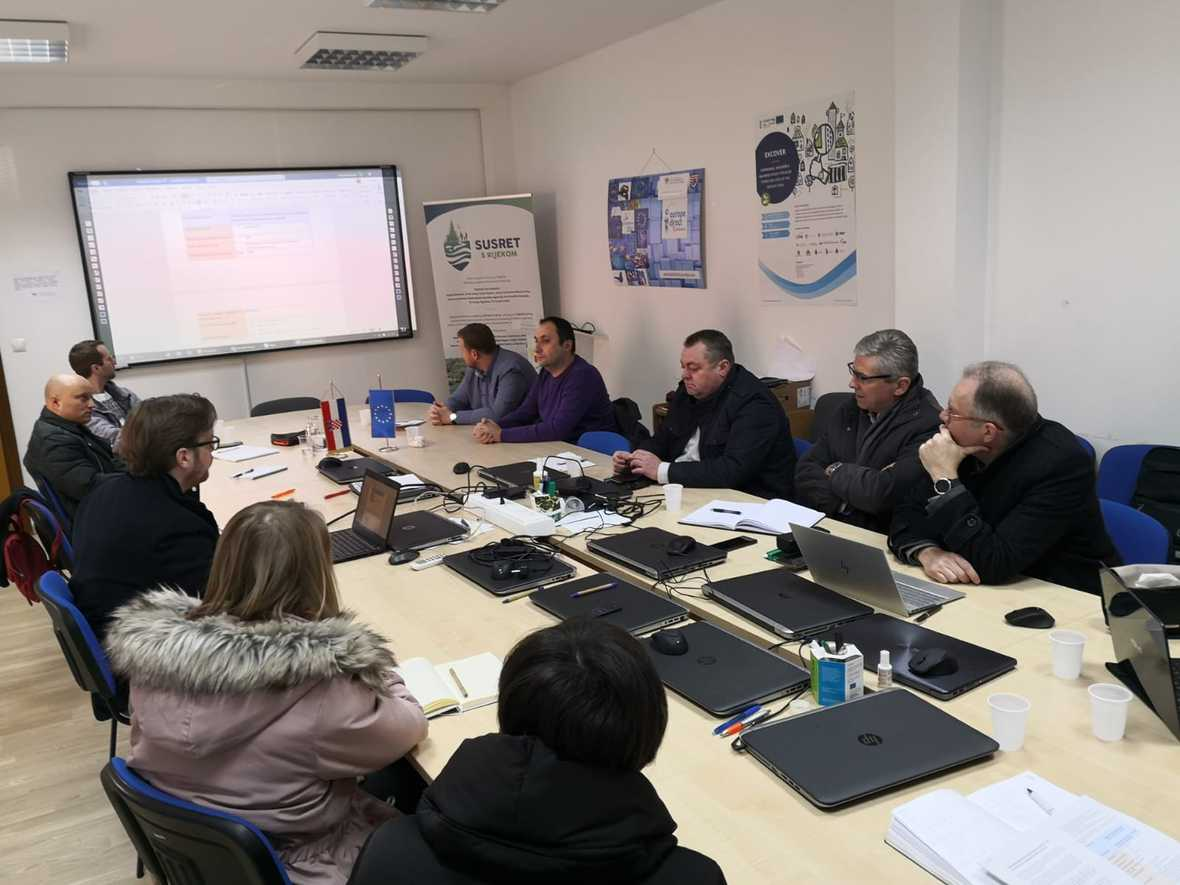Like   Tweet   in

## New SECAPs, Upcoming Events, and an updated website...

 /* styles */ While a lot has changed in the world since our last newsletter in February, the PentaHelix project has continued to develop climate action plans with the engagement of all stakeholders. This cohesive policy development is the subject of our upcoming online EUSEW side-event and features a presentation from WVI who recently submitted SECAPs for eight municipalities in Flanders, Belgium! Find out more about the event and other news below! About PentaHelix: The project aims to empower local and regional authorities to find innovative and cost-effective approaches to develop, finance, implement, and improve Sustainable Energy and Climate Action Plans (SECAPs) that contribute to reaching national and European climate and energy goals and policies.
 /* styles */ If you want to be regularly updated and receive the latest information, you can register to our newsletter, follow us on Twitter, LinkedIn, or Facebook, or get involved in our climate forum!
 table div table+table+table+table+table div table{width:100%;padding:0}table div table+table+table+table+table div table img{width:96.23%;padding:0;float:none}table div table+table+table+table+table div table td{width:100%;padding:0 1.88% 18px}/* styles */## New SECAPs from WVIMarch 2020, Belgium | SECAPs for the 8 municipalities of ‘Klimaatoverleg Midwest’ have been finalised and submitted. The Commission will now evaluate whether the plan meets the requirements. However, it goes without saying that the municipalities do not need to wait for the official approval to put the proposed measures into practice. More information about the measures they have taken and how they are taking the lead in achieving concrete climate ambitions can be found here.

 table div table+table+table+table+table+table+table+table+table div table{width:100%;padding:0}table div table+table+table+table+table+table+table+table+table div table img{width:96.23%;padding:0;float:none}table div table+table+table+table+table+table+table+table+table div table td{width:100%;padding:0 1.88% 18px}/* styles */table div table+table+table+table+table+table+table+table+table+table+table div table,table.module-10{width:48.87%;float:left;padding:0}table div table+table+table+table+table+table+table+table+table+table+table div table a{border:0 none;text-decoration:none}table div table+table+table+table+table+table+table+table+table+table+table div table img{width:100%!important;border:0 none;text-decoration:none}table div table+table+table+table+table+table+table+table+table+table+table div table td{width:100%;padding:0 20px 20px 0}/* styles */ ‘Energising the European Green Deal: Engaging stakeholders in multi-level governance’. We are very happy to announce that we will be hosting an online event alongside EUSEW on 18 June at 10:00 – 11:30 CET. Our session will assess initiatives happening at the local, regional, and EU level to highlight synergies and barriers to comprehensive and cohesive energy policy in the EU. Featuring speakers from PentaHelix, and other EU projects COLEOPTER, COMETS, PAC, and PlanUP. Find out more and register HERE to make sure you don’t miss it!
 table div table+table+table+table+table+table+table+table+table+table+table+table div table{width:100%;padding:0}table div table+table+table+table+table+table+table+table+table+table+table+table div table img{width:96.23%;padding:0;float:none}table div table+table+table+table+table+table+table+table+table+table+table+table div table td{width:100%;padding:0 1.88% 18px}/* styles */PentaHelix will be hosting an online roundtable with MEPs on Monday 22nd June 13:00 – 14:30 CEST. We will have partners from across Europe join the event with the aim to translate the normal European Parliament event style into the digital space, with MEPs and institution representatives joining roundtables with stakeholders to discuss key questions on accelerating the transition in Europe and how we can work better together. We look forward to meeting many of you on Monday 22nd June for an exciting online event. For more information and to register to join the event then please click here.

 table div table+table+table+table+table+table+table+table+table+table+table+table+table+table+table div table{width:100%;padding:0}table div table+table+table+table+table+table+table+table+table+table+table+table+table+table+table div table img{width:96.23%;padding:0;float:none}table div table+table+table+table+table+table+table+table+table+table+table+table+table+table+table div table td{width:100%;padding:0 1.88% 18px}/* styles */## Updates to our Climate Forum and websiteA number of updates have been made to the PentaHelix website since December and here are the two that we're most excited about:

▪ Our Climate Forum is now open-view, so you only need to sign in to comment!
▪ Brand new interactive maps of PentaHelix pilots and replicators! Check them out here!
 ▪ Our Climate Forum is now open-view, so you only need to sign in to comment!
 ▪ Brand new interactive maps of PentaHelix pilots and replicators! Check them out here!
 table div table+table+table+table+table+table+table+table+table+table+table+table+table+table+table+table+table+table div table{width:100%;padding:0}table div table+table+table+table+table+table+table+table+table+table+table+table+table+table+table+table+table+table div table img{width:96.23%;padding:0;float:none}table div table+table+table+table+table+table+table+table+table+table+table+table+table+table+table+table+table+table div table td{width:100%;padding:0 1.88% 18px}/* styles */No longer just on Twitter, you can now find PentaHelix on Facebook and LinkedIn too! We have been sharing our updates and related news across all three platforms and on pentahelix.eu, so be sure to give a follow to stay updated!

 table div table+table+table+table+table+table+table+table+table+table+table+table+table+table+table+table+table+table+table+table+table div table{width:100%;padding:0}table div table+table+table+table+table+table+table+table+table+table+table+table+table+table+table+table+table+table+table+table+table div table img{width:96.23%;padding:0;float:none}table div table+table+table+table+table+table+table+table+table+table+table+table+table+table+table+table+table+table+table+table+table div table td{width:100%;padding:0 1.88% 18px}/* styles */## 'European Green Deal will be at the heart of’ Post-COVID Europe

 table div table+table+table+table+table+table+table+table+table+table+table+table+table+table+table+table+table+table+table+table+table+table+table div table,table.module-22{width:47.17%;float:right;padding:0}table div table+table+table+table+table+table+table+table+table+table+table+table+table+table+table+table+table+table+table+table+table+table+table div table a{border:0 none;text-decoration:none}table div table+table+table+table+table+table+table+table+table+table+table+table+table+table+table+table+table+table+table+table+table+table+table div table img{width:100%!important;border:0 none;text-decoration:none}table div table+table+table+table+table+table+table+table+table+table+table+table+table+table+table+table+table+table+table+table+table+table+table div table td{width:100%;padding:0 0 20px 20px}/* styles */ EU Commissioner for Energy, Kadri Simson, announced in May that national energy and climate plans should play a key role in the recovery of the EU. She also stated that now is an ideal opportunity to enhance the progress towards the EU’s climate neutrality goal. Learn more about PentaHelix and the European Green Deal here!
 table div table+table+table+table+table+table+table+table+table+table+table+table+table+table+table+table+table+table+table+table+table+table+table+table div table{width:100%;padding:0}table div table+table+table+table+table+table+table+table+table+table+table+table+table+table+table+table+table+table+table+table+table+table+table+table div table img{width:96.23%;padding:0;float:none}table div table+table+table+table+table+table+table+table+table+table+table+table+table+table+table+table+table+table+table+table+table+table+table+table div table td{width:100%;padding:0 1.88% 18px}/* styles */## €10,000 available for innovative sustainable actions through Transformative Action Award

 table div table+table+table+table+table+table+table+table+table+table+table+table+table+table+table+table+table+table+table+table+table+table+table+table+table+table div table,table.module-25{width:43.77%;float:left;padding:0}table div table+table+table+table+table+table+table+table+table+table+table+table+table+table+table+table+table+table+table+table+table+table+table+table+table+table div table a{border:0 none;text-decoration:none}table div table+table+table+table+table+table+table+table+table+table+table+table+table+table+table+table+table+table+table+table+table+table+table+table+table+table div table img{width:100%!important;border:0 none;text-decoration:none}table div table+table+table+table+table+table+table+table+table+table+table+table+table+table+table+table+table+table+table+table+table+table+table+table+table+table div table td{width:100%;padding:0 20px 20px 0}/* styles */ Transformative Action Award applications are OPEN! If you are a city/region/civil organisation you can win 10,000€ to kick-start an action in your area! Find more information & apply here by 31st July 2020. If you would like to find out more about financing climate actions in particular, check out the ‘Financing’ discussion on our Climate Forum.
 table div table+table+table+table+table+table+table+table+table+table+table+table+table+table+table+table+table+table+table+table+table+table+table+table+table+table+table div table{width:100%;padding:0}table div table+table+table+table+table+table+table+table+table+table+table+table+table+table+table+table+table+table+table+table+table+table+table+table+table+table+table div table img{width:96.23%;padding:0;float:none}table div table+table+table+table+table+table+table+table+table+table+table+table+table+table+table+table+table+table+table+table+table+table+table+table+table+table+table div table td{width:100%;padding:0 1.88% 18px}/* styles */## Be active in Sustainable Energy and Climate Action - Join the Climate Forum!

PentaHelix wants everyone to have the opportunity actively participate in discussions on Sustainable Energy and Climate Action and we want to hear from you on our Climate Forum!

## PentaHelix Online Support Tools

On our website, there are free resources available to aid SECAP development including:

And more!

## Climate Communication Guidelines available on the Website

Climate Communication Guidelines developed from Østfold’s Climate Communication Workshop are now available in English, Spanish, Norwegian, Dutch, Croatian, and Latvian on our website.

 table div table+table+table+table+table+table+table+table+table+table+table+table+table+table+table+table+table+table+table+table+table+table+table+table+table+table+table+table+table+table+table+table+table+table div table{width:100%;padding:0}table div table+table+table+table+table+table+table+table+table+table+table+table+table+table+table+table+table+table+table+table+table+table+table+table+table+table+table+table+table+table+table+table+table+table div table img{width:96.23%;padding:0;float:none}table div table+table+table+table+table+table+table+table+table+table+table+table+table+table+table+table+table+table+table+table+table+table+table+table+table+table+table+table+table+table+table+table+table+table div table td{width:100%;padding:0 1.88% 18px}/* styles */